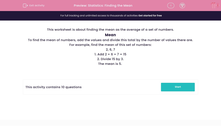# Statistics: Finding the Mean

In this worksheet, students calculate the mean of a given set of data.Key stage:  KS 2

Curriculum topic:   Maths and Numerical Reasoning

Curriculum subtopic:   Mean, Median, Mode and Range

Difficulty level:#### Worksheet Overview

This worksheet is about finding the mean as the average of a set of numbers.

Mean

To find the mean of numbers, add the values and divide this total by the number of values there are.

For example, find the mean of this set of numbers:

2, 6, 7

1. Add 2 + 6 + 7 = 15

2. Divide 15 by 3.

The mean is 5.

### What is EdPlace?

We're your National Curriculum aligned online education content provider helping each child succeed in English, maths and science from year 1 to GCSE. With an EdPlace account you’ll be able to track and measure progress, helping each child achieve their best. We build confidence and attainment by personalising each child’s learning at a level that suits them.

Get started